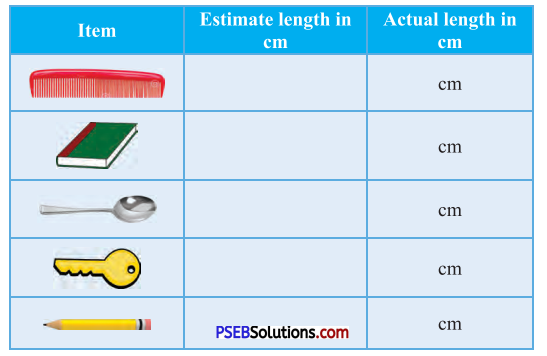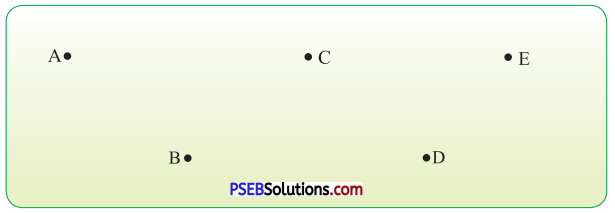# PSEB 4th Class Maths Solutions Chapter 5 Measurement Ex 5.1

Punjab State Board PSEB 4th Class Maths Book Solutions Chapter 5 Measurement Ex 5.1 Textbook Exercise Questions and Answers.

## PSEB Solutions for Class 4 Maths Chapter 5 Measurement Ex 5.1

Question 1.
Complete the tableSolution:
Take the above mentioned items and measure each of them and complete the table.Question 2.
Find the distance between given dots and give the following answers:(a) Distance from point A to B
= ……… cm
Solution:
4 cm

(b) Distance from point B to D
= ……… cm
Solution:
6 cm

(c) Distance from point A to E
= ……… cm
Solution:
5 cm

(d) Distance from point C to D
= ……… cm
Solution:
4 cm

(e) Distance from point B to E
= …….. cm
Solution:
11 cm

(f) Distance from point A to D
= …….. cm
Solution:
6 cm.